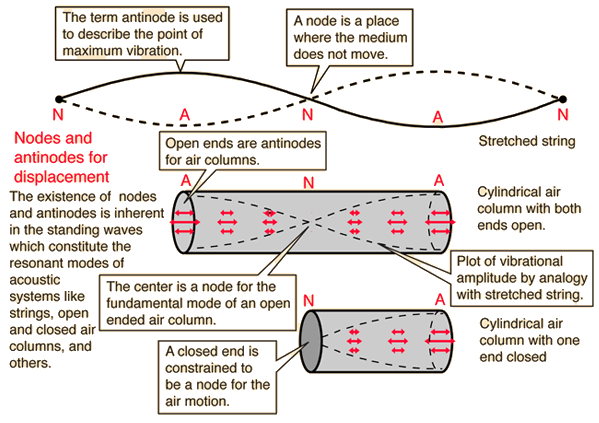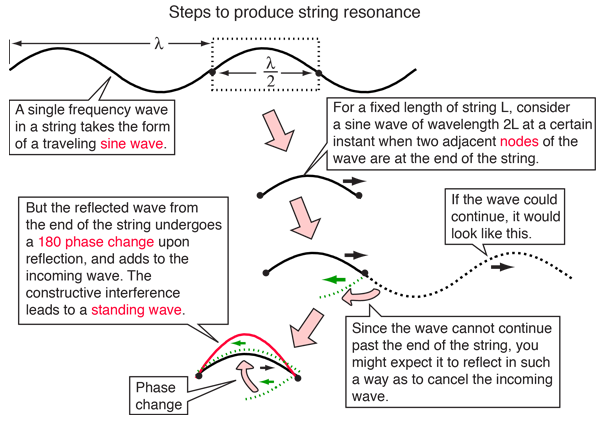# Fundamental and Harmonics

The lowest resonant frequency of a vibrating object is called its fundamental frequency. Most vibrating objects have more than one resonant frequency and those used in musical instruments typically vibrate at harmonics of the fundamental. A harmonic is defined as an integer (whole number) multiple of the fundamental frequency. Vibrating strings, open cylindrical air columns, and conical air columns will vibrate at all harmonics of the fundamental. Cylinders with one end closed will vibrate with only odd harmonics of the fundamental. Vibrating membranes typically produce vibrations at harmonics, but also have some resonant frequencies which are not harmonics. It is for this class of vibrators that the term overtone becomes useful - they are said to have some non-harmonic overtones.

The nth harmonic = n x the fundamental frequency.

 Harmonics in cents
Index

Wave concepts

Resonance concepts

 HyperPhysics***** Sound R Nave
Go Back

# Sinusoidal Waves

A single- frequency traveling wave will take the form of a sine wave as a function of distance. The top sine wave in the illustration below is such a sine wave, a transverse wave typical of that caused by a small pebble dropped into a still pool.The bottom sine wave in the illustration above is a sine wave as a function of time. Such a wave would be produced by plotting the position vs time of a mass on a spring undergoing simple harmonic motion. Or, if the mass on the spring is carried at a constant speed across a room, its position would trace out a sine wave as a function of distance.

Sine waves can be represented mathematically and it can be shown that any wave can be constructed from an appropriate combination of sine waves (Fourier synthesis).

Index

Wave concepts

Resonance concepts

 HyperPhysics***** Sound R Nave
Go Back

# Nodes and Antinodes

The standing waves produced by wave motion in strings or air columns can be used to establish the values for wavelength, frequency and speed for the waves in accordance with the wave relationship, v = fλ.Displacement vs pressure in standing wave
Index

Wave concepts

Resonance concepts

 HyperPhysics***** Sound R Nave
Go Back

# Steps to Produce String ResonanceYou may click on the highlighted text for further detail.
Index

Wave concepts

Resonance concepts

 HyperPhysics***** Sound R Nave
Go Back